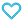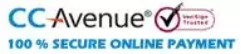# WB JEE Chapterwise Explorer Mathematics-Engineering

500.00

MTG’s WB JEE Chapter-wise Explorer – Mathematics is outlined with chapter-wise theory based on most recent syllabus. It consists of category wise exercises and previous 5 years (2017-2021) questions to get a clear insight of questions asked in the exam. For a better practice of upcoming exam 3, Model test papers with detailed solutions are provided.

96 in stock

Qty:Add to WishlistMTG WB JEE Chapter-wise Explorer – Mathematics is an essential book designed for all aspiring engineer appearing for WB JEE engineering entrance exam. The book comprehensively covers the syllabus of WB JEE Mathematics and provide pointwise theory,
formulas and important theorem for each Chapter to fulfil the urge of aspirants. The book consists of category wise practice exercises and previous 5 years (2017-2021) questions of WB JEE entrance exam. For a better practice of upcoming exam 3, Model test papers with
detailed solutions are provided. Wb JEE Mathematics provides a clear insight of questions asked in the previous years’ examination

• 1. A.P., G.P., H.P.
• 2. Logarithms
• 3. Complex Numbers
• 5. Permutation and Combination
• 6. Principle of Mathematical Induction
• 7. Binomial Theorem
• 8. Matrices and Determinants
• 9  Sets, Relation and Mappings
• 10. Statistics and Probability
• 11. Trigonometric Functions
• 12. Solution of Triangles
• 13. Inverse Trignometric Functions
• 14. Straight Lines
• 15. Circle
• 16. Conic Sections
• 17. Limits and Continuity
• 18. Derivatives
• 19. Application of Derivatives
• 20. Indefinite Integrals
• 21. Definite Integrals
• 22. Application of Integrals
• 23. Differential Equations
• 24. Three Dimensional Geometry
• 25. Vector Algebra
• Model Test Papers
• Model Test Paper 1
• Model Test Paper 2
• Model Test Paper 3
ISBN11 9789391191610 MTG Editorial Board 2022-23 404 Class 11, Class 12 Regional Ent. Exams-Engg Previous Years Papers 604gm

## Reviews

There are no reviews yet.